#Function Repository Resource:

# HypergraphPlot

Plot a hypergraph defined by a list of hyperedges

Contributed by: Jaebum Jung
 ResourceFunction["HypergraphPlot"][{{vi1,vi2,…},…}] generates a plot of the hypergraph with hyperedges {{vi1,vi2,…},…}.

## Details and Options

Hyperedges can be any expression.
The following options are supported:
 "BaseLayout" "SpringEmbedding" how to lay out vertices "SubsetBoundary" Automatic whether to draw the boundary of subsets "SubsetBoundaryScale" 2 scale factor of the subset boundary size "SubsetBoundaryStyle" Automatic styles for subsets "SubsetEdge" Automatic whether to draw edges connecting vertices in subsets "SubsetEdgeStyle" styles for subset edges "IndexLabeled" overall theme for vertices labels and placements for vertices style to use for vertex labels generate graphic shapes for vertices 0.15 size of vertices styles for vertices

## Examples

### Basic Examples

Draw a hypergraph:

 In:=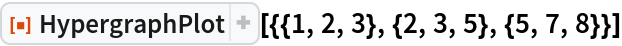Out=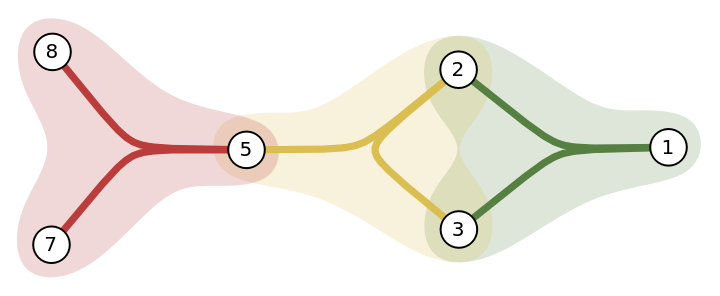### Scope

Draw a hypergraph without edges connecting subsets:

 In:=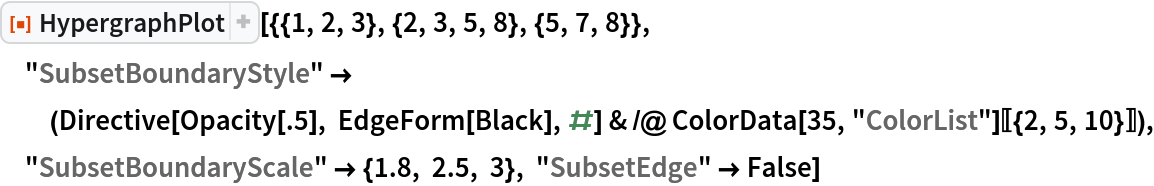Out=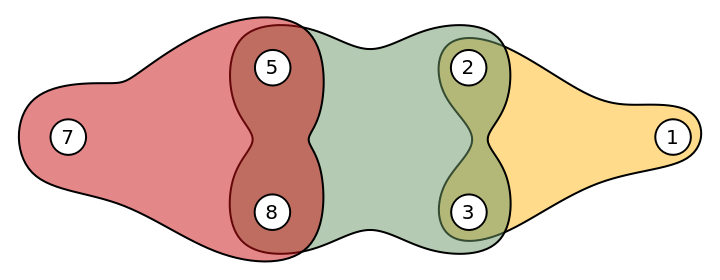Draw a hypergraph with different vertex styles:

 In:=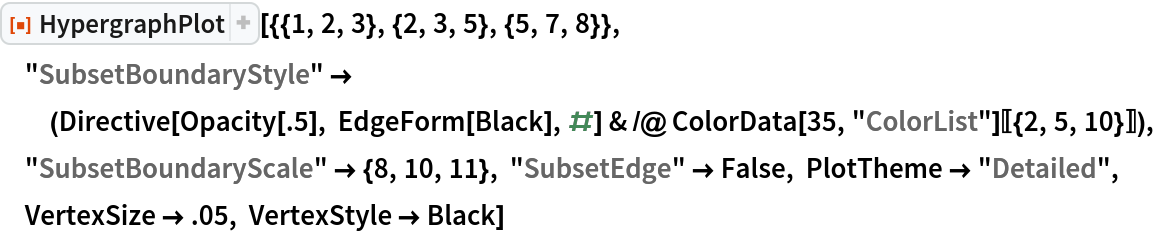Out=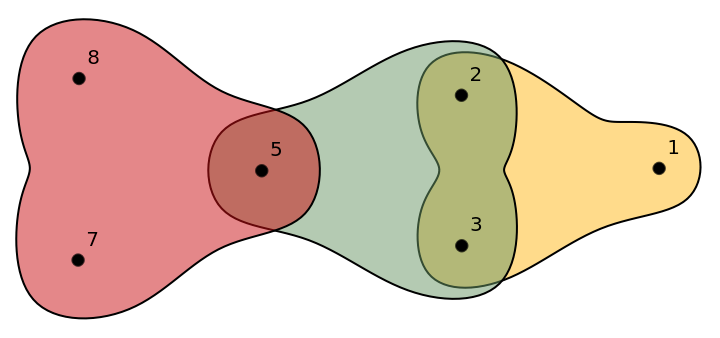### Options

#### BaseLayout

The default base layout is "SpringEmbedding":

 In:=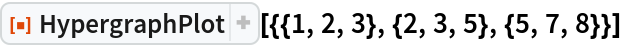Out=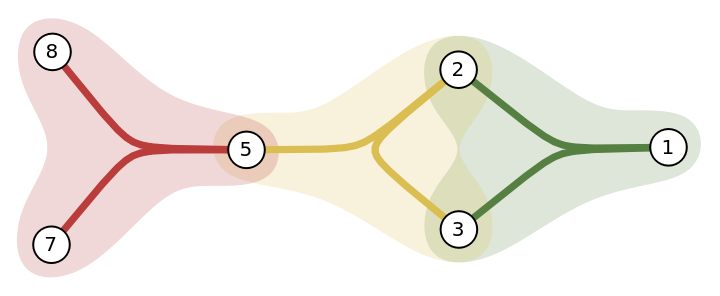In:=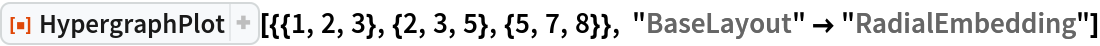Out=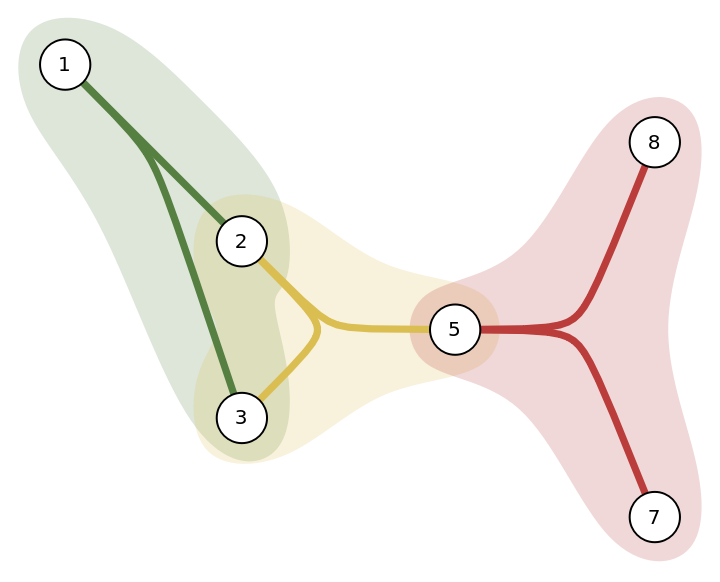#### SubsetBoundary

The default subset boundary is Automatic:

 In:=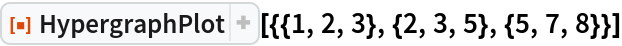Out=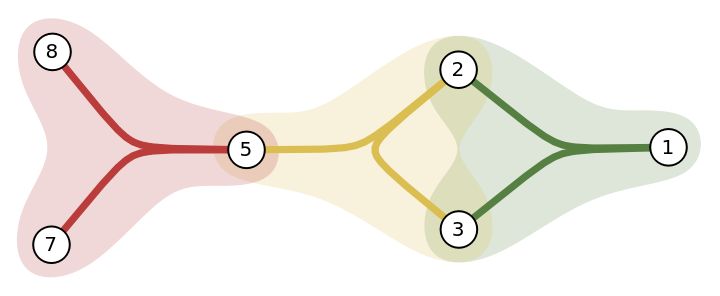Use False to not show subset boundaries:

 In:=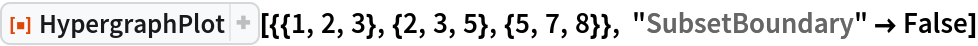Out=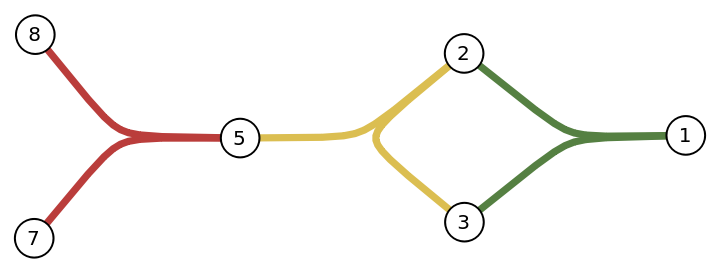Alternate between True and False:

 In:=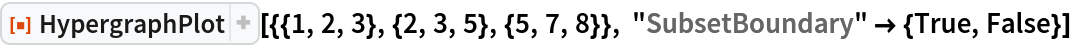Out=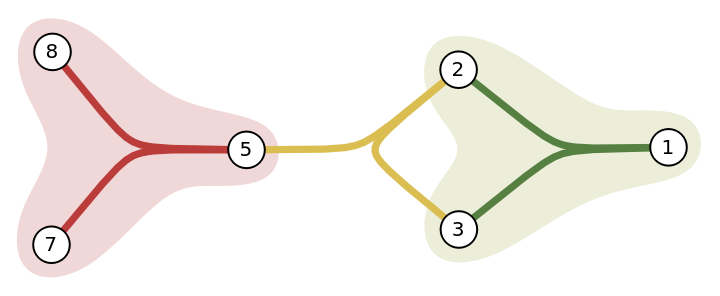#### SubsetBoundaryScale

The default subset boundary scale factor is 2:

 In:=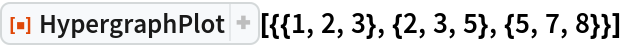Out=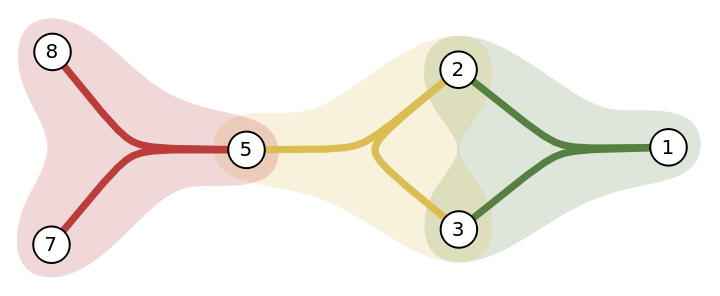Increase the scale factor to 3:

 In:=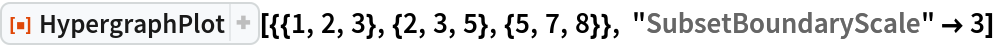Out=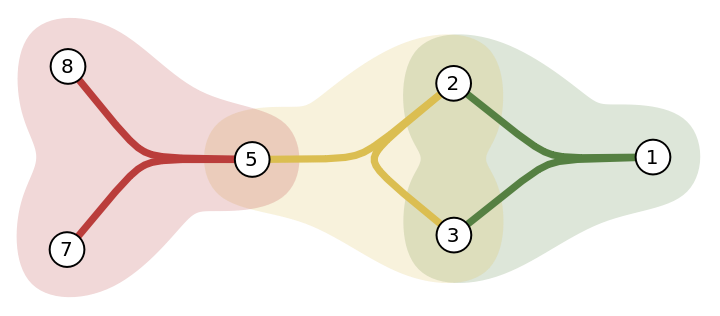Alternate between 3 and 5:

 In:=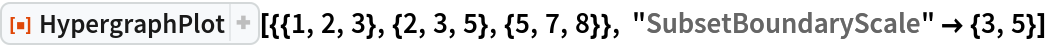Out=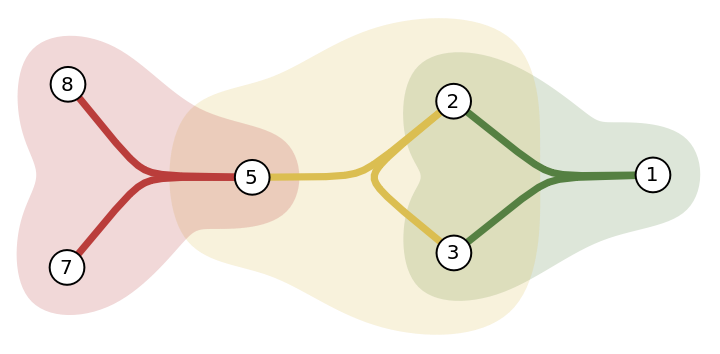#### SubsetBoundaryStyle

The default subset boundary style is Automatic:

 In:=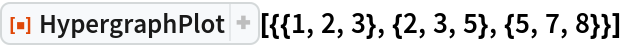Out=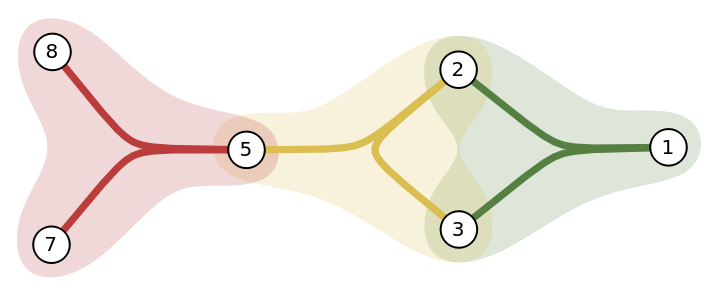Use black boundary lines:

 In:=Out=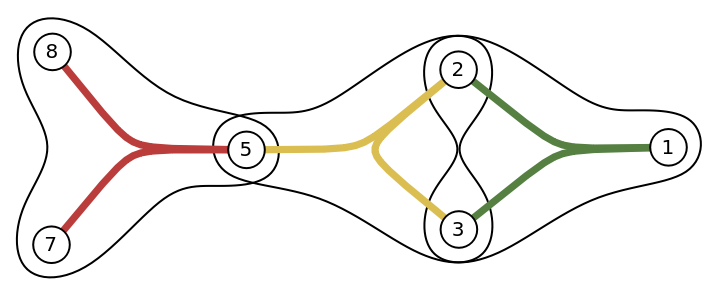Alternate between red and blue:

 In:=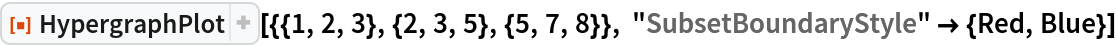Out=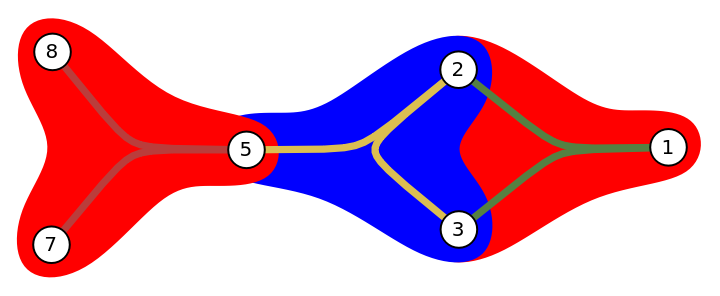#### SubsetEdge

The default subset edge is Automatic:

 In:=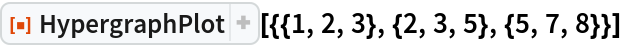Out=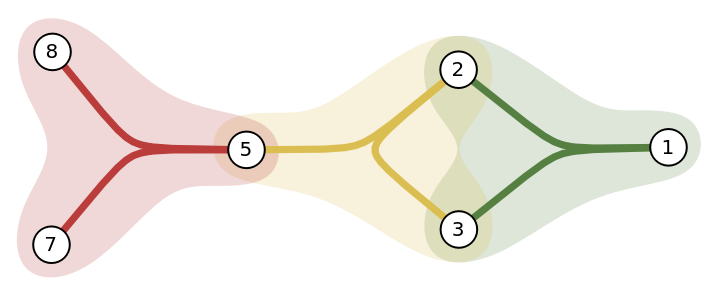Use False to not show subset edges:

 In:=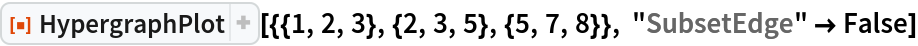Out=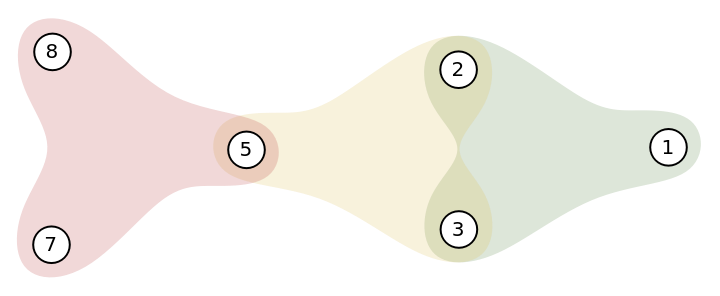Alternate between True and False:

 In:=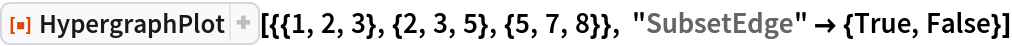Out=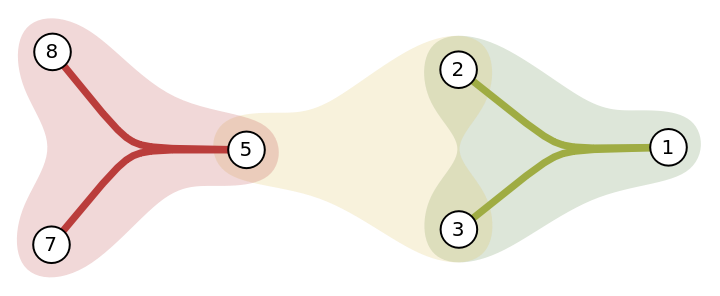#### SubsetEdgeStyle

The default subset edge style is Automatic:

 In:=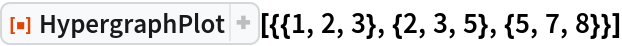Out=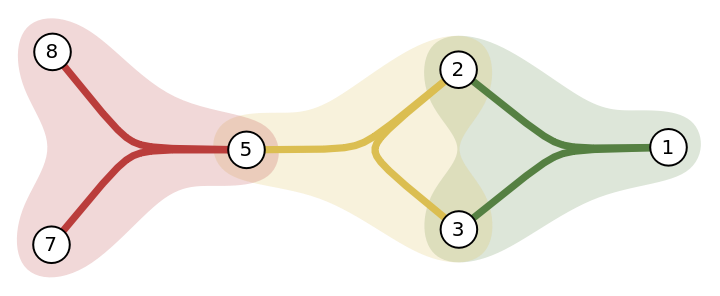Use black edge lines:

 In:=Out=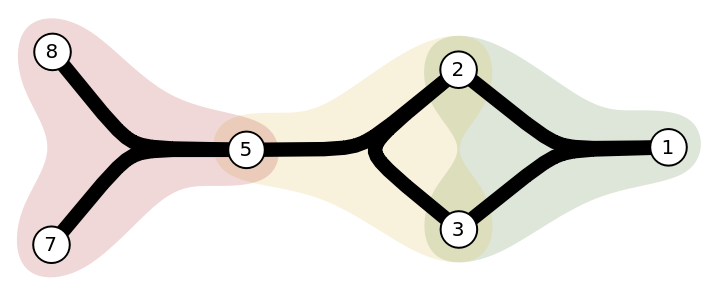Alternate between red and blue:

 In:=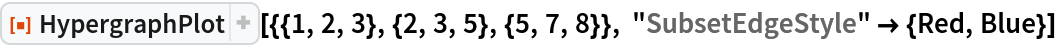Out=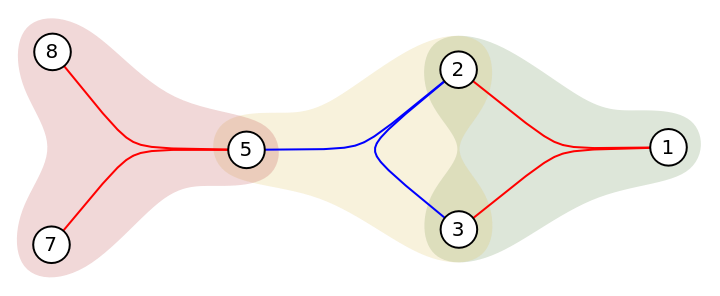### Applications

Draw a hypergraph constructed by communities:

 In:=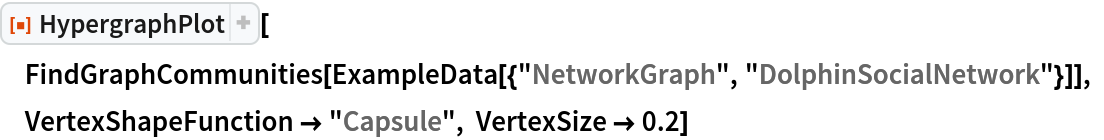Out=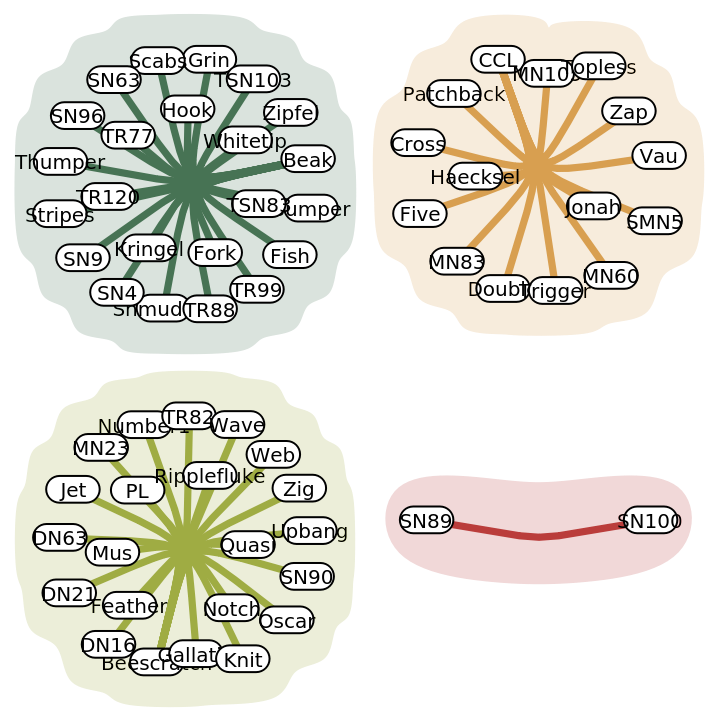Pick the two most involved members of the terrorist network:

 In:=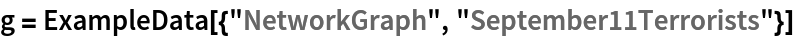Out=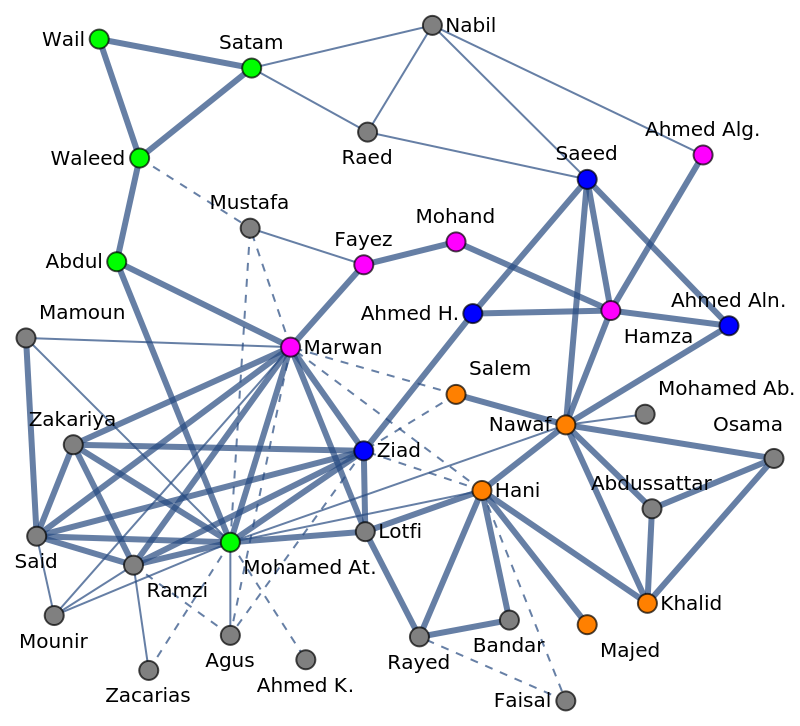In:=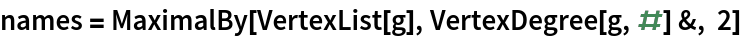Out=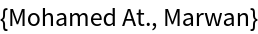Generate a hypergraph by members connected to most involved members:

 In:=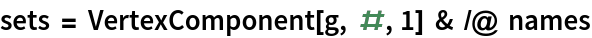Out=Show a hypergraph:

 In:=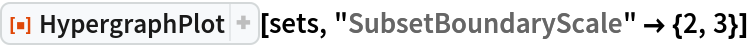Out=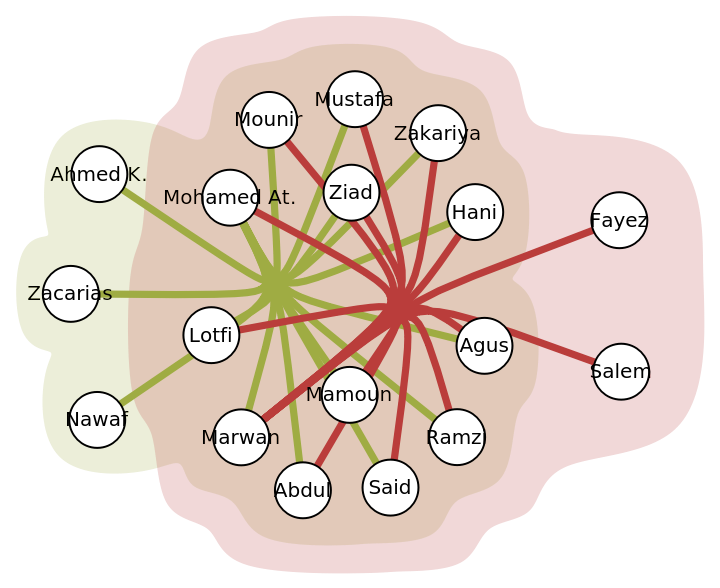## Author Notes

A prior function under this name was moved to the new name SimpleHypergraphPlot.